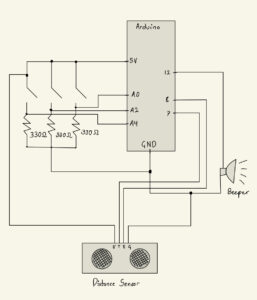# Home Work 10 – Sarthak and Yerk (AutoTune)

## AutoTune

### Concept:

For this project, we were inspired by AutoTune. AutoTune allows you to take a certain musical key and make sure all the notes are aligned within that key, thereby perfecting the sound.

### Execution:

For the circuit, we used a buzzer, three switches, and a distance sensor. Initially, we used the key of C major and the note C as our reference point. Having calculated the mathematic relationships that exist between notes in a major key, we assigned different intervals of distance to different notes for that key. For instance, if the user’s hand is 0-5 cm away from the sensor, the circuit will play a C note. If the hand is 5-10 cm away, the circuit will play a D note. This method does not let notes that do not belong in the C major key to be played, which perfects the sound of the board. Just like AutoTune.

When the yellow button is pressed, the key that is being played is transposed up a half step. So if the user is playing in a C major key, once the yellow button is pressed, the user will now play in D flat major. The green button executes the opposite effect – it transposes the key down a half step. If a user is playing in F major, upon pressing the button, they will play in E major.

Finally, if the blue button is pressed, the user will now play in the natural minor key, completely changing the mathematical relationships that underlie the notes. For instance, if the user was playing in the G major key, once they press the button, they will hear the G minor key. This process goes the other way around: if the button is pressed again, the user will go back to the major key.

###Code:

```int note_c = 262;

const int beeperPin = 12;
const int trigPin = 7;
const int echoPin = 8;
const int yellowSwitch = A0;
const int greenSwitch = A2;
const int blueSwitch = A4;

const int division = 5;

int flag = 1;

// this is the number of notes in an octave
const int num = 8;

int all_notes[num];

int getDistance() {
// this bit of code was taken from https://howtomechatronics.com/tutorials/arduino/ultrasonic-sensor-hc-sr04/

// calculating the distance between the user and the sensor
digitalWrite(trigPin, LOW);
delayMicroseconds(2);

digitalWrite(trigPin, HIGH);
delayMicroseconds(10);
digitalWrite(trigPin, LOW);

int duration = pulseIn(echoPin, HIGH);
int distance = duration * 0.034/2;

Serial.print("Distance: ");

return distance;
}

int getNote(int distance) {
int index = distance / division;

return all_notes[index];
}

void makeMajorKey() {
// making an array of all the notes in an octave given in the major key.
all_notes = note_c;
int n = 1;
for (int i = 1; i < num; i++) {
// the third and seventh note change is a half step.
if ((n % 3 == 0 && n % 6 != 0) || n % 7 == 0){
all_notes[i] = all_notes[i-1] * pow(2, 1.0/12.0);
} else {
all_notes[i] = all_notes[i-1] * pow(2, 1.0/6.0);
}
n++;
}
}

void makeMinorKey() {
// making an array of all the notes in an octave in the minor key.
all_notes = note_c;
int n = 1;
for (int i = 1; i < num; i++) {
// the second and fifth note change is a half step.
if ((n % 2 == 0 && n % 4 != 0 && n % 6 != 0 && n % 8 != 0) || n % 5 == 0){
all_notes[i] = all_notes[i-1] * pow(2, 1.0/12.0);
} else {
all_notes[i] = all_notes[i-1] * pow(2, 1.0/6.0);
}
n++;
}
}

void setup() {
pinMode(beeperPin, OUTPUT);
pinMode(trigPin, OUTPUT);
pinMode(echoPin, INPUT);
pinMode(yellowSwitch, INPUT);

Serial.begin(9600);

makeMajorKey();
}

void loop() {
// PipeLine
// get the distance of an object
int distance = getDistance();
if (distance <= division * num) {
// create note for that distance
int note_to_play = getNote(distance);

// play the note
tone(beeperPin, note_to_play);

// switch to transpose up

// switch to transpose down

// switch to toggle between major and natural minor key

if (yellow_state == HIGH) {
// transposing the key of the instrument a half-step up, when the switch is clicked.
note_c = note_c * pow(2, 1.0/12.0);
makeMajorKey();
}

if (green_state == HIGH) {
// transposing the key of the instrument a half-step down, when the switch is clicked.
note_c = note_c / pow(2, 1.0/12.0);
makeMajorKey();
}

// changes major key to natural minor key and vice versa.
if (blue_state == HIGH) {
if (flag == 1) {
makeMinorKey();
flag = 0;
} else {
makeMajorKey();
flag = 1;
}
}
}
}```

### Future Improvements:

One of the issues with the current board is that the code may register the clicking of the button as multiple clicks, even if the user had pressed the button once. With the yellow and the green buttons, this leads to a higher or a lower transposition than expected. With the blue button, there are instances when it simply skips over the shift to the minor key because it registers one click as two. In the future, we want to work toward eliminating that inconvenience.